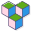CppBuzz# Java program to find factorial

``````public class Main {

public static void main(String[] args) {
//!5 = 5*4*3*2*1 = 120

int num = 5;
int fact = 1;

for(int i=1; i<= num; i++) {
fact = fact*i;
}

System.out.println("Factorial of " + num + " is : " + fact);
}

}``````